﻿ Forecast Performance of Multiplicative Seasonal Arima Model: an Application to Naira/Us Dollar Exchange Rate

### Forecast Performance of Multiplicative Seasonal Arima Model: an Application to Naira/Us Dollar Excha...

ONASANYA OLANREWAJU. K, OLUSEGUN ALFRED OLAKUNLE, ADENIJI OYEBIMPE EMMANUELOPEN ACCESSPEER-REVIEWED

## Forecast Performance of Multiplicative Seasonal Arima Model: an Application to Naira/Us Dollar Exchange Rate

ONASANYA OLANREWAJU. K1,, OLUSEGUN ALFRED OLAKUNLE1, ADENIJI OYEBIMPE EMMANUEL1

1Department of Statistics, University of Ibadan, Nigeria

### Abstract

Seasonality accounts majorly for quarterly and monthly movements in macro-economics and time series and in modelling such patterns to have a forecasting robustness, precision and reliability have been steadily increased in recent years but no consensus has been reached as to which model yields the appropriate description. However, this research seeks to address the robustness and accuracy of seasonal ARIMA model of Naira/Dollar exchange rate using a monthly data for the period of Jan 1994 to July 2013. The series was subjected to a time plot revealing seasonal patterns and upward trend. Both behaviours were taken into account by moving average and seasonal moving average, where little or fewer months of data were used to estimate the local level and trend and also few seasons of data were averaged over to estimate the seasonal patterns. The proposed model shows predictive power, robustness and precision which was revealed by the normality of data and residual analysis. The estimated forecast values from our proposed model are more realistic and closely reflect in the current economic reality in two countries as indicated by the forecast measurement criteria.

### At a glance: Figures

123
Prev Next

• K, ONASANYA OLANREWAJU., OLUSEGUN ALFRED OLAKUNLE, and ADENIJI OYEBIMPE EMMANUEL. "Forecast Performance of Multiplicative Seasonal Arima Model: an Application to Naira/Us Dollar Exchange Rate." American Journal of Applied Mathematics and Statistics 2.3 (2014): 172-178.
• K, O. O. , OLAKUNLE, O. A. , & EMMANUEL, A. O. (2014). Forecast Performance of Multiplicative Seasonal Arima Model: an Application to Naira/Us Dollar Exchange Rate. American Journal of Applied Mathematics and Statistics, 2(3), 172-178.
• K, ONASANYA OLANREWAJU., OLUSEGUN ALFRED OLAKUNLE, and ADENIJI OYEBIMPE EMMANUEL. "Forecast Performance of Multiplicative Seasonal Arima Model: an Application to Naira/Us Dollar Exchange Rate." American Journal of Applied Mathematics and Statistics 2, no. 3 (2014): 172-178.

 Import into BibTeX Import into EndNote Import into RefMan Import into RefWorks

### 1. Introduction

Seasonality has been a major check in time series majorly in business and economic time series. Seasonality reveals itself when there is no periodicity. Seasonality in time series is a regular pattern of changes that repeats itself over S time period, where S defines the number of time periods until the regular pattern changes. Seasonality occurs in monthly data in which high values tend always to occur in some particular months and low values tends to always to occur in other particular months. In this case, S=12 (months per year) is the span of the periodic seasonal behaviour, for quarterly data S=4. Using the span of seasonality, one can predict the current month value using Box Jenkins (1976), for example a seasonal first order autoregressive model would use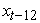to predict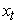, for second order autoregressive would useand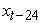to predict. However, seasonality often accounts for major part in quarterly and monthly movement in some macro- economic time series, and modelling and forecasting of such patterns has been steadily increased in recent years, but no consensus has been revealed as to which model yields the appropriate description. The most popular model used in seasonality in the literature are (i) seasonal with unit root (ii) model in first and second difference (iii) periodic models (iv) models with a constant. Osborn (2001), then Franses and Paap (2001) have studied the relative performance of these models. However, this research entails to justify whether seasonal ARIMA model has a predictive/ forecasting power to predict economic time series future values (out sample forecast) and within sample in foreign market usage and these will be judge under the instrument of the measurement criteria mean square error, mean absolute error and mean absolute percentage error. The analysis will be carried out in the software use of E-view 6.0. The remaining part of this paper is section as follows: section 2 covers the data source, theory and methodology, section 3 discusses the results and lastly section 4 focuses on conclusions and possible recommendation.

### 2. Data source, Theory and Methodology

2.1. Data Source

In carrying out this research, a monthly time series data on Nigeria exchange rate (naira against the US dollar) for period from January 1994 to July 2013 was collected from the website www.oanda.com. This data has two components, the dependent variable and independent variable. The dependent variable is the exchange rate while the independent variable is the time and time component is in months. Figure 1 shows the time plot of the series which aids to know the presence of outliers and the judge for Stationarity using E-view 6.0.

Figure 1. The plot of Naira/dollar exchange rate for the period Jan 1994 to Jul 2013
2.2. Theory

Box Jenkins (1976), Chatfield (1975), and Brillinger (2008) in the literature have discussed several procedures in building ARIMA modelling. The seasonal ARIMA incorporates both seasonal and non-seasonal factors in multiplicative model. The shorthand notation of the model is: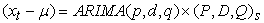(1)

Where p = non-seasonal AR orderP = seasonal AR order q = non-seasonal MA orderQ = seasonal MA order d = non-seasonal differencing D =seasonal differencing, s = time span of repeating seasonal patterns.

Without any differencing operators, the Eq (1) could be written more formally as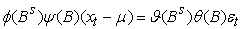(2)

The non-seasonal components are: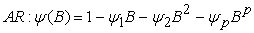(3)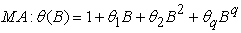(4)

The seasonal components are: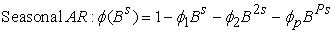(5)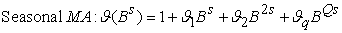(6)

Where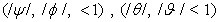satisfies the Stationarity and invertibility conditions. Also each coefficient is defined as the root of the characteristics equation (3-6).

Mere looking at Eq. (2), the seasonal and non-seasonal AR component multiply each other at the left and side, the seasonal and non-seasonal MA component multiply each other at the other side which shows that it incorporates both seasonal and non-seasonal factors.

2.3. Methodology

To carry out the aim of this research, we encompass a time plot which aids in describing the pattern and general behaviour of the series. The series was examined for Stationarity, outliers and other interventions. Since our data is a monthly data, we look straight in the time plot and found if there is any seasonal pattern:

If there is seasonality and no trend, then a difference of lag (S) is taken (12th month differencing).

If there is linear trend and no obvious seasonality, a first difference will make it Stationary or if otherwise, a curve trend exhibits, a proper transformation of the series is needed.

If there is both trend and seasonality, a non-seasonal difference and a seasonal difference is applied to the data as two successive operators, which means we are to examine the ACF and PACF of :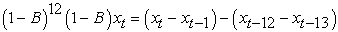(7)

Where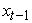= one lag time period of= naira/dollar exchange rate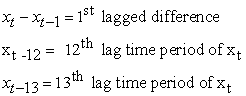To determine the ACF and the PACF of Eq. (7), it requires a lot of guessing. This guessing will be used in choosing the seasonal and non-seasonal orders of AR and MA.

For non-seasonal terms: we examine the earliest lags (1, 2, and 3) to judge non-seasonal terms. Spikes in the ACF (at low lags) indicates the non-seasonal MA terms, also spikes in the PACF (at low lags) indicates possible non-seasonal AR terms.

For seasonal terms: we examine the patterns across lags that are multiple of S = 12 months (12, 24, 36). Spikes in the ACF (at lag 12, 24) indicates seasonal MA terms, likewise also spikes in the PACF (at lag 12, 24) indicates seasonal AR terms.

Next is to build the seasonal ARIMA in which we are to estimate the parameters. The parameters of seasonal ARIMA always tend to be small but depend on large observations (sample points).

2.3.1. Model Diagnosis

To check for the adequacy of the estimated seasonal ARIMA model, the fitted model is subjected to model diagnosis by the LM test, R2, and the appropriate model is determined by the forecasting criteria. The error term is expected to normally distributed i. e identically distributed. We check this by testing the null hypothesis of white noise residual. Following the Ljung Box (1978), the variance of the autocorrelation is given by: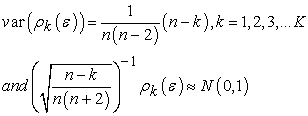(8)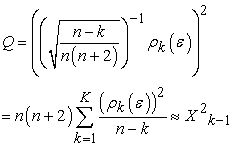(9)

where K-1=k-p-q and p+q does not include in the constant tern.

For LM test of serial correlation, we test for AR (p) errors in: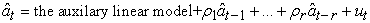(10)

where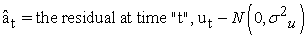.

The null hypothesis is: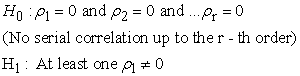(11)

Test statistics: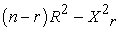. Note, no obvious answer as reached in determining the value of (r) in the empirical studies but for AR, ARMA: r = p+1 lags, for seasonal r = s, where s is the span of periodicity.

A further test for normality is judged by plotting the ACF of residual of the estimated model, if the ACF hovers around the line then the model is adequate; hence a trivial solution or the model built in Eq. (2) is revised. When the model is adequate, forecast is implemented.

Forecast of seasonal ARIMA are computed for both in sample and out sample values. The optimum forecast value are evaluated using the mean squared error (MSE) which measures the average of squared error over the sample period, mean percentage error (MPE) which measures the average of percentage error by which forecast differs from outcomes, mean absolute error which measures the average of absolute error dollar amount or percentage points by which forecast differs from outcome.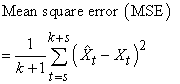(12)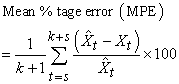(13)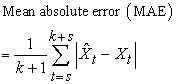(14)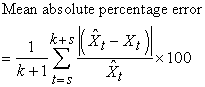(15)

Where t = s, 1+s, …, k + s, and the actual and predicted value for corresponding t values are denoted by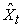and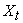respectively.

The smaller value of Eq (12), Eq (13), Eq (14) and Eq (15) gives the best seasonal model.

### 3. Discussion and Results

Figure 3. Autocorrelation function and Partial autocorrelation function values at lag 55 of the differenced series for the period Jan 1994 to July 2013. *** Indicates that there are spikes at the lags 1, 12, 13 under the ACF and likewise also at lags 1, 12, 24, 36 and 48 under PACF and these can be easily seen in figure 4

Following the figure 1 which shows the time plot of Naira/US Dollar exchange rate, indicates that there is some kind of non-Stationarity and a possible non-linearity in the observed data. Further observations shows that there is an upward trend from the period of 2000 till 2003 and further maintain stable pattern till 2007, depreciated in 2008, maintain stability till 2009 and rising up in year 2009 till August 2013. The fluctuation in the observed data points across the period indicates the presence of seasonal patterns. To remove such trends and seasonality a first difference and 12th seasonal difference was applied to the original data series respectively. Figure 2 below shows the graph of the differences applied.

From the graph above, it shows that seasonal pattern has been depressed to an extent and the mean reversion of the data’s at each point is constant over time after taking account of the differences. There is just an indication of peak in the year 1995 and trough in the year 1997 and which is reasonable to an extent. Since there is control of seasonality and trend, we moved ahead and take account on seasonal and non – seasonal terms. To determine the seasonal terms and non – seasonal terms we plot the autocorrelation values against the lags taken account on the differenced series. We had 222 observations, 55 lags was examined according to Gujarati (2006) on the maximum lag selection. Figure 3 and Figure 4 displays the ACF, PACF values, and the plot of correlogram, partial correlogram respectively.

Figure 4. (The plot of Autocorrelation function and Partial autocorrelation function up to lag 55 of the differenced series for the period Jan 1994 to July 2013 sd_d_rate means the seasonal difference of the first difference of naira/dollar exchange rate

Following Figure 4 above under the ACF, we can see that there is a spike at lag 1, its value is more than zero but it is significant. We can add an MA (1) to account for the non – seasonal MA terms. Considering the multiples lags of seasonal period (12) i.e. (12,24,36,48) lags under , it shows that only at lag 12 shows a spike or autocorrelation and the rest of the lags hovers around the line, hence we can add SMA (1) to account for the seasonal MA terms. Under the PACF at lag 1, there is a spike where its PACF is greater than zero but also significant. Hence we can add an AR (1) to account for the non – seasonal AR terms. Mere looking at multiple lags of seasonal period (12) i.e. (12, 24, 36, 48) under PACF, it shows that there are spikes at each lags which accounts for seasonal AR (terms). Since we have spikes at lag 12, 24, 36, and 48 under PACF, hence we compared the following seasonal ARIMA models: ARIMA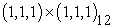, ARIMA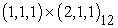, ARIMA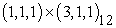, ARIMA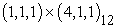and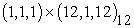and the best model was chosen based on the forecast measurement criteria. Table 1 displays the model summary.

#### Table 1. Model summaryDownload asPowerPoint Slide

Veiw figureView current table in a new window

The forecast evaluation was based on the dynamic forecasting with the implementation of back casting MA (terms).

The asterisk indicates that based on the smallest value of measurement criteria, seasonal ARIMAis the best model.

The proposed model is: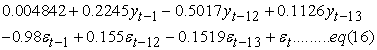Check appendix for the derivation of the above equation.

From the estimated model in Figure 5, we introduced constant in the model since we had first difference, seasonal difference in filtering the trend and controlling the seasonal patterns respectively. The constant doesn’t have much significant information on explaining the dependent variable (Naira/US Dollar exchange rate). On the validity of the coefficient of parameters, all the coefficients are valid (less than one) and they are all significant since their corresponding p – values is less than 0.05, 0.01 level of significance, which conforms to Box Jenkins (1976) i.e. the parameters satisfy the Stationarity and invertibility conditions by falling in the unit circle, hence to some extent the naira/us dollar exchange rate is covariance stationary. The inverted AR and MA roots which were obtained from Eq (3) and Eq (4) (lag polynomials) is less than one, hence these justify that the too much of over difference was not examined, the coefficient of AR, MA can be interpreted and can be used for forecasting of the dependent variable (naira/us dollar) and these result conforms to Hamilton (1994). The AR (1) and SAR (12) introduced indicates that we can predict the current naira/dollar rate of any month using its past month. The MA (1) and SMA (12) in the estimated model reflect to smoothing and estimating the seasonal patterns. A small value in MA (1) coefficient suggest that little smoothing is being needed to estimate the local level and trend or few months of data is needed to estimate the local level and trend. From the estimated model MA (1) is -0.98, the value is so small in such that little or fewer months of data are used in estimating the local level and trend. The coefficient of SMA (12) is 0.155 which is also small, this means that little or small seasons of data are being averaged over to estimate the seasonal pattern. To judge for the appropriateness of this model we compare the value of R2 and the Durbin Watson statistics. As we can see the R2 = 0.60949 is less than Durbin statistics = 1.919996, hence the model is appropriate and which conforms to Granger and New bold (1986). For the overall parameter significance, as we can see that Prob (F – statistics) = 0.00000 is less than the exact or observed probability (0.05) which indicates that we reject the association of no relationship of parameters to the Naira/Dollar exchange rate and conclude that lagged of the dependent (Naira/Dollar rate), error lagged values are used in forecasting the current (naira/dollar exchange. Since our model is adequate, we move ahead to perform the residual analysis (diagnostic checking). Figure 6 displays the LM test for serial correlations in the residual obtain in the estimated model of equation (16).

It can be seen that serial correlation is not present among the errors since the probability of having a large value of F – statistic = 1.5698 is greater than the observed probabilities (0.01, 0.05), hence we conclude that the seasonal ARIMAis adequate and be simply be efficient in carrying out prediction and forecasting. We forecasted for in and out sample forecast using both dynamic and static forecasting procedure. Dynamic forecasting as the usage of calculating dynamic, and multiple step ahead of forecasting using the first period in the forecast sample but depends on the dynamic components; lagged dependent variable and ARMA terms. Static forecasting has the ability to forecast for sequence of one step- ahead forecast using the actual rather than the forecasted values of the dependent variable. Since we have these, Figure 7 and Figure 8 displays the graph of the In and Out sample forecast at level form of the dependent variable (Naira/ US Dollar exchange rate) using dynamic and static forecast respectively.

The Thiel’s inequality coefficient (0.557934) indicates that the dynamic forecasting method do not show a greater forecasting accuracy since its value is largely different from zero.

Theil inequality coefficient value close to 0 indicates a forecasting accuracy, hence as in Figure 8, the Thiel’s inequality coefficient (0.015674) indicates that the static forecasting method shows a greater forecasting accuracy since its value is not largely different from zero. In summary of the forecast values obtained from static forecast, Figure 9 displays the comparison between the actual, forecasted and the residual starting from 2012:01 to 2013:08.

Figure 9. Comparison between the actual, forecast values from 2012:01 to 2013:08

From figure 9, it appears that the seasonal ARIMAhave predictive power, more efficient and hence the best model to describe the structure behaviour of the series (Naira/dollar exchange rate) which aid in control of stock and foreign exchange market movement. We checked oanda website which deals with world exchange rate data on all updated economic indicators for August 2013 average naira/dollar rate and it was found out that the average naira/dollar exchange rate for August 2013 was 159.5659 and our forecasted value obtained from our proposed model was 159.8603 which is indicated by the asterisk. The good result we obtained as justify the major aim of this research and hence we conclude that the seasonal ARIMA model proposed is good and accurate model for forecasting economic and time series data that exhibit seasonal behaviour.

### 4 .Conclusion

The main aim of this research is to justify whether the seasonal ARIMA model has a predictive power or forecasting ability to predict economic time series data which exhibit seasonal variation or behaviour. The aim is subjected to the methodology of the good work of Box and Jenkins (1976). The time plot, which is the first step to examining some hidden characteristics reveals non-stationarity (and upward trend) and seasonal patterns. To control the seasonal patterns and trend, a 12th seasonal difference and a first difference method were applied respectively and a partial autocorrelation function, the autocorrelation function partly implies the addition of AR(1), MA(1), SAR(1) and SMA(1) which all together built a seasonal ARIMA. The coefficient of MA (1), and SMA (1) of the estimated model proposed shows that few or little month of data are been used to estimate the local level and trend, and also few or fewer months of season of data are being average over to estimate the seasonal patterns. The proposed seasonal model showed flexibility, predictive accuracy and power based on the residual analysis which shows normality in data and presence of no serial correlation. The estimated forecasted values from our proposed model are much more realistic and closely reflect current economic reality in the two countries as indicated by the forecast measurement criteria.

This research therefore recommends that a seasonal ARIMA model of this type is exploited by decision and policy makers, forecasters and researchers who make use of economic time series data which exhibits seasonal behaviour. Data exploration should also be properly scrutinized before carrying out time series data analysis because it endeavours to reveal the hidden characteristics of the series.

### References

  Box, G.E.P and Jenkins, G.M. (1976). Time series Analysis: Forecasting and Control, Revised Edition, Holden-Day.In article  Brockwell, P.J. and Davis, R. (2002). A. Introduction to Time series and Forecasting. Springer-Verlag, New York.In article CrossRef  Brillinger, M. et. al (2008). Time series data Analysis and Theory Expanded Edition, Mc-Graw-Hill Inc. New York.In article  Chatfield, C. (2003). The Analysis of Time series: An introduction. Chapman and Hall, London.In article  Damodar N.G. & Dawn C.P (2006). Basic Econometrics. M.C. Graw Hill.In article  Dickey, D.A and Fuller, W.A. (1979). Distribution of the estimators for Autoregressive time series with a unit root. Journal of the American Statistical Association. 74: 427-431.In article  Ghysels, E. and Osborn, D.R. The Econometric Analysis of Seasonal Time series. Cambridge, U.K: Cambridge University.In article  E Ghysels, Osborn, D.R, Rodrigues, P. (2006). Forecasting Seasonal Time series, in Handbook of Economic Forecasting. Vol. 1, ed. Elliot, G., Granger, C. and Timmermann, A. pp: 659-711. Elsevier.In article CrossRef  Hamilton, J. (1994). Time series Analysis. Princeton, New Jersey: Princeton University Press.In article  Hooper, P and J. Martins (1982): Fluctuations in the dollar: A model of nominal and real exchange rate determination”. Journal of International Money and Finance. 1: 39-56.In article CrossRef  Ljung, G.M and G.E.P Box (1978). On a Measure of lack of fit in time series models. Biometrika. 65: 297-303.In article CrossRef  Mills, T.C (1999). The Econometric Modeling of Financial Time series. Cambridge University Press.In article CrossRef  Pfaff, B (2004). Urca “Unit root and Cointegration test for time series” R Package, version 0, 6-1.In article  Theil, H. (1966). Applied Economic Forecasting. North-Holland, Amsterdam.In article

### APPENDIX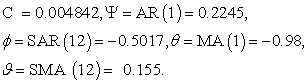The general equation is: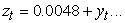(17)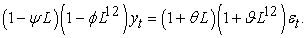(18)

Opening the brackets, we have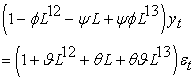(19)

Opening the brackets: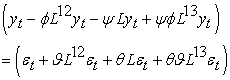(20)

Using the backshift operator to covert the lag polynomials.

Recall that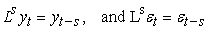Then we have;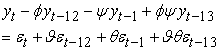(21)

Re –arranging: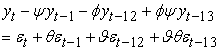(22)

Making yt the subject of formula, we have: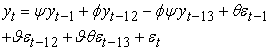(23)

Now substituting the coefficient of the parameters: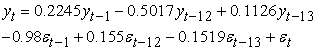(24)

The estimated model of Eq (17) is re –writing as: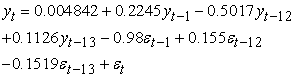(25)